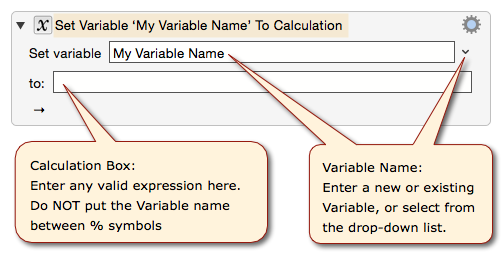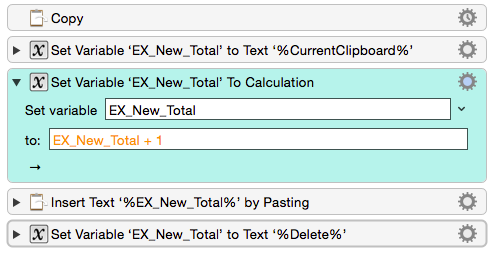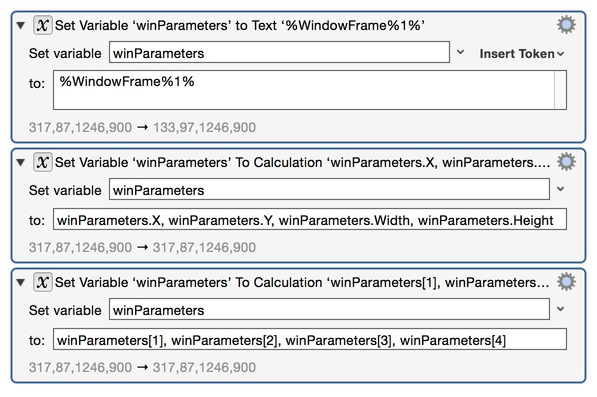#Keyboard Maestro Wiki

### Site Tools

action:Set_Variable_to_Calculation# Set Variable to Calculation Action

The Set Variable to Calculation action sets a variable to the result of a calculation with numeric formatting.## Calculations

You may use the following in the to calculation box:

The to field is a numeric field so you cannot use any of the Clipboard tokens or indeed any text tokens, except within a CALCULATE function.

## Variables

The to field is a numeric field, so you use variable names unadorned:

• Do not use the text token syntax for a Variable, like `%Variable%My Variable Name%`.
• Instead, you just use the Variable name without any `%`, like `My Variable Name`

You can use the Edit ➤ Insert Function menu to insert functions.

## Formatting

As can be seen by the blue shade of the action gear menu there is an additional option for the action, specifically you can Format the Result using Unicode Number Format Patterns such as #,##0.##.

## Result

At the bottom of the action you can see the current value of the calculation.

If the calculation is invalidly formatted, the invalid part of the calculation will show up in RED.

If the calculation would currently fail because an input variable does not currently have a numeric value, that variable will show up in ORANGE.

## Examples

### Example 1

The below macro performs a simple calculation of adding 1 to the value currently selected by the user in the user's document, and then pasting the new value back into the user's document.### Example 2### Forum